# Valid expressions

The string template variable supports the use of valid expressions, which can embed variables, random data, and the result of mathematical operations. This guide provides an overview of the different types of valid expressions that are supported by the string template variable:

# Valid expression syntax

Valid expressions are delimited by double curly braces: {{ }}. Variables are also prepended by an @ symbol. Here are some examples of valid expressions:

• `{{@username}}`: references the value of a variable `username`
• `{{alpha:6}}`: generates a random string of six upper and lower case letters
• `{{fake.name.firstName}}`: generates a random first name
• `{{(6 + 10) / 2}}`: divides the sum of six and ten by two

# Referencing variables

Using mabl variable syntax, you can embed a variable within a string template variable.

### 📘Mabl variable syntax

The syntax for referencing variables in mabl is {{@variable_name}}. For example, if you want to reference a variable called "user_id", you can write `{{@user_id}}`

In the Pattern text field, type the `{{@variable_name}}` in the spot where you want to add the original variable. The Current value box will show a preview of your new variable.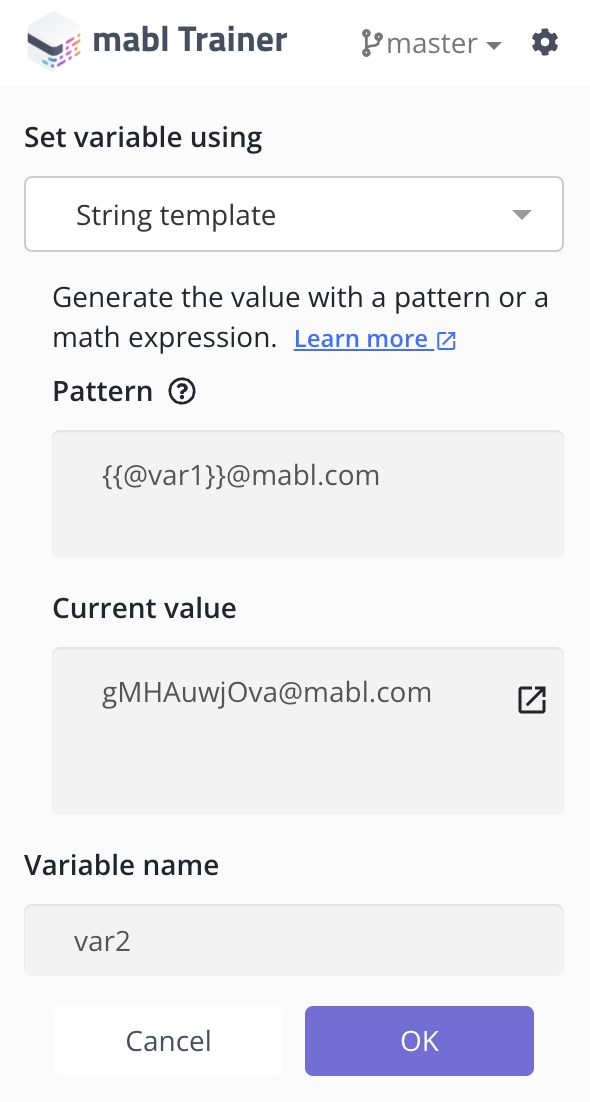Give the new variable a name and click OK .

### 📘Limitations to referencing variables

Variable interpolation is not supported in data-driven variables or environment variables. This means that their values cannot contain references to another variable, such as `{{@anotherVar}}`.

# Macros

Macros are useful for generating random strings. They include a character type followed by a colon and a number, where the number indicates the number of random characters to be created. Here is the table of supported macros that can be used for string templates:

Macro

Description

Example

`alpha`

Upper and lower case letters

`{{alpha:3}}`

`alphaLower`

Lowercase letters (a-z)

`abc{{alphaLower:4}}`

`alphaUpper`

Uppercase letters (A-Z)

`ABC{{alphaUpper:5}}`

`digit`

Numbers (0-9)

`123-{{digit:4}}`

`alnum`

Alphanumeric (letters and numbers)

`random{{alnum:6}}`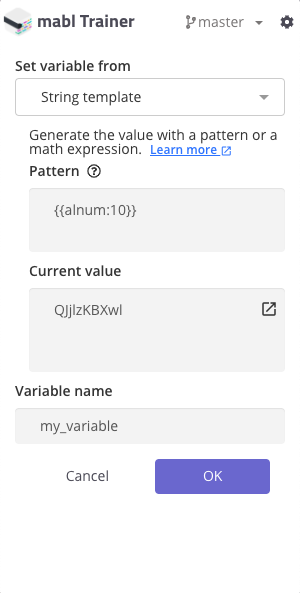# Random data

Mabl can also generate realistic random data using the string template. The random data expressions have to be enclosed in double curly braces and have to be prepended by "fake.". Mabl expects the expression to be in the form {{fake.type.subtype}}. For example, to generate a random last name, you can use `{{fake.name.lastName}}`. Below are some examples of how to use the string template to create random data.

Random monetary values can also be generated as well:

Even random titles can be created: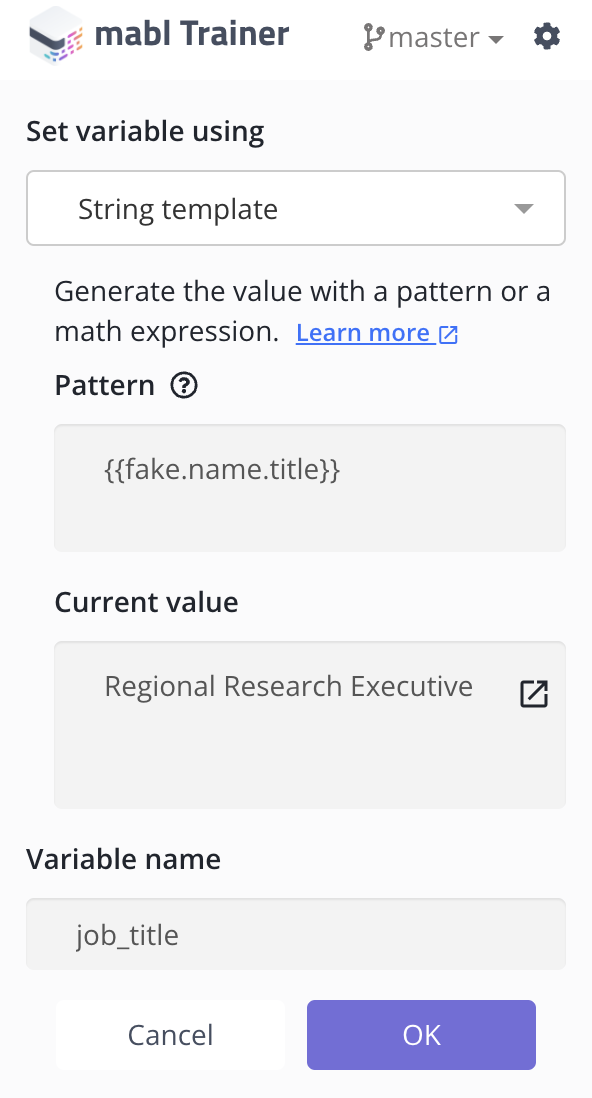Below are some of the more commonly used expressions for creating random data:

Expression

Expectation

Sample Result

`{{fake.name.firstName}}`

Random first name

"Garfield"

`{{fake.name.lastName}}`

Random last name

"Larson"

`{{fake.address.streetAddress}}`

"840 Roberts Burg"

`{{fake.address.city}}`

Random city name

"Manteton"

`{{fake.address.state}}`

Random US state name

"North Carolina"

`{{fake.address.zipCode}}`

Random US zip code

"02160"

`{{fake.address.countryCode}}`

Random 2-letter country code

"TN"

`{{fake.company.companyName}}`

Random company name

"Sawayn Group"

`{{fake.phone.phoneNumber}}`

Random phone number in one of multiple formats

"1-968-628-9831 x738"

`{{fake.phone.phoneNumberFormat}}`

Random phone number in the format ###-###-####

"776-705-5652"

`{{fake.lorem.text}}`

Random filler text

"consequatur omnis neque"

`{{fake.random.uuid}}`

Random UUID

"71bcc5d3-7f24-4b08-bcf8-f9b8e0d6d913"

`{{fake.finance.amount}}`

Random financial amount

"17.78"

### 📘Note

Only the English locale is supported.

# Valid mathematical expressions

Mabl can perform basic math operations within the string template and save the results to a new variable or overwrite an existing variable. Formulating the expression is important for the correct results. The mathematical expressions must be enclosed by the double curly braces to be evaluated. Here are examples of how to create valid math expressions in the string template:

Math expressions will be evaluated under the PEMDAS rules.

• Example: `{{5 + 3 / 2}}` will evaluate to 6.5

Use parentheses to evaluate math operations in the specified order.

• Example: `{{(5 + 3) / 2}}` will evaluate to 4

Combine math operations with a "\$" or "%" symbol:

• Example: `\${{(5 + 3 ) / 2}}` will evaluate to \$4

Use macros and variables (must be a number) in math operations.

• The following screenshot shows an example using a digit macro within a math calculation. This will evaluate the macro (2) and add 100 to the macro.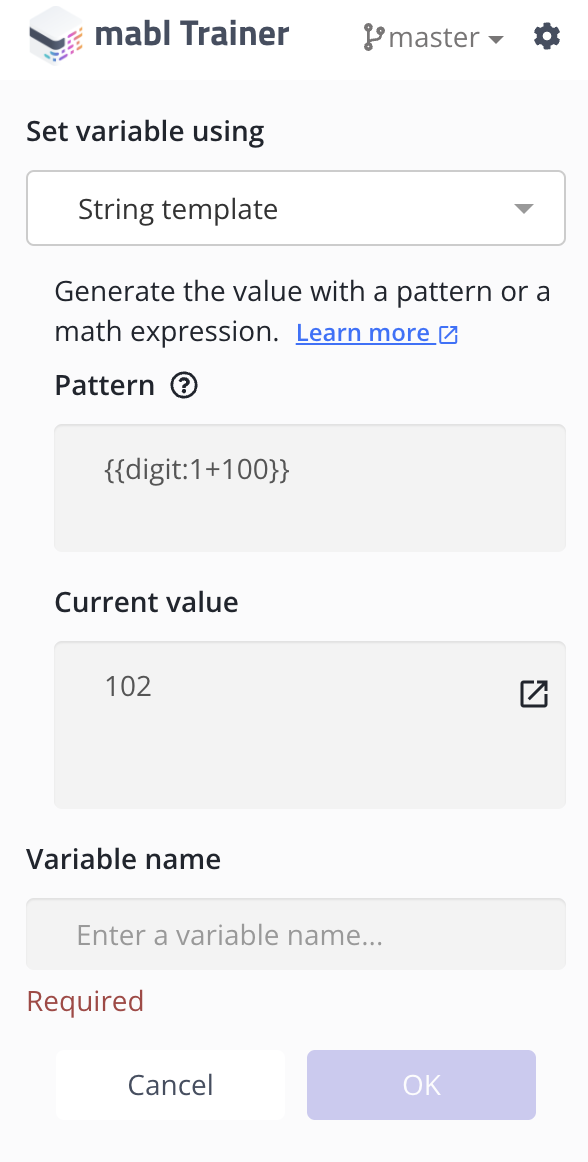• If the value of a variable is a number, you can include it in a math operation. Consider the example in the following screenshot, where the variable `number` is divided by 2: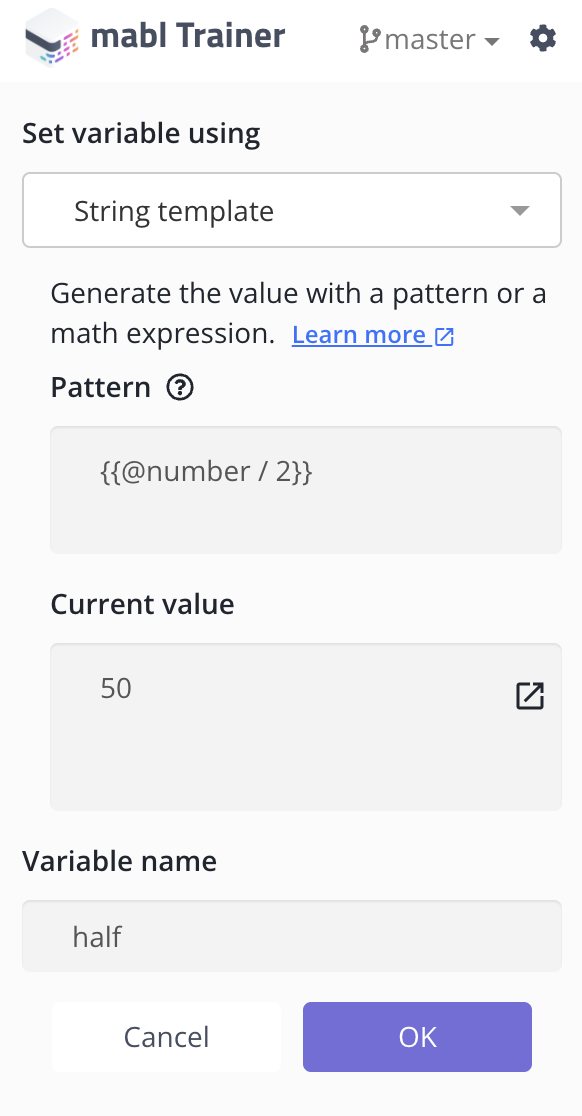# Advanced math expressions

Mabl also supports certain math functions to format the numerical result from a math expression. Below are the functions that are supported:

1. `round(number, numberOfDecialPlaces)` - Rounds the result of a math calculation to `numberOfDecialPlaces` number of decimal places.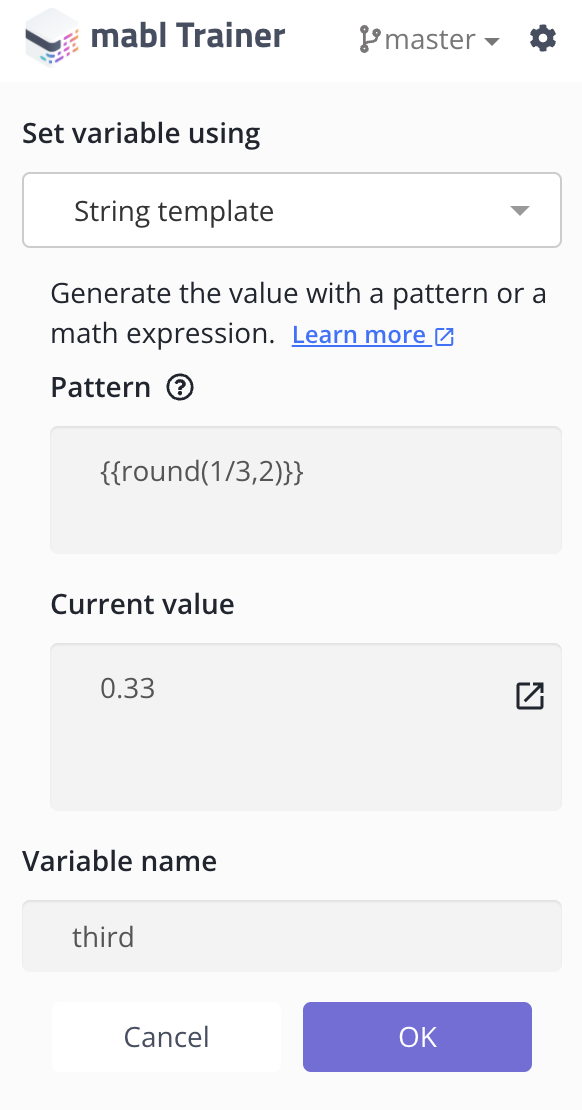### 🚧JavaScript Floating Point Precision

Due to rounding errors stemming from how floating point numbers are stored, arithmetic expressions like `0.1 + 0.2 === 0.3` will return `false`. To resolve this, the result needs to be rounded, e.g. `round(0.1 + 0.2, 1)` or `(0.1 + 0.2).toFixed(1)`.

1. `abs(number)` - Returns the absolute value of a math calculation
Example: `{{abs(-6)}}` will evaluate to `6`

2. `randomInt(min, max)` - Returns a random integer greater than or equal to `min` but LESS than `max`
Example: `{{randomInt(0, 11)}}` will return a random integer between 0-10.